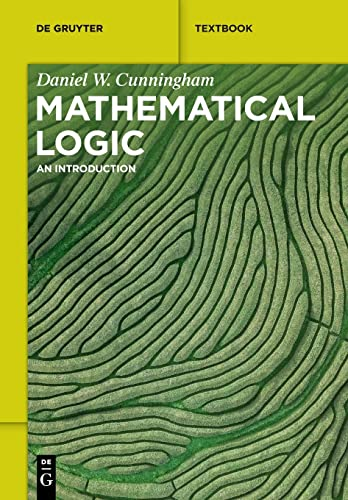# Mathematical Logic: An Introduction

• Length: 305 pages
• Edition: 1
• Publisher:
• Publication Date: 2023-06-20
• ISBN-10: 3110782014
• ISBN-13: 9783110782011
Description

Mathematical Logic: An Introduction is a textbook that uses mathematical tools to investigate mathematics itself. In particular, the concepts of proof and truth are examined.

The book presents the fundamental topics in mathematical logic and presents clear and complete proofs throughout the text. Such proofs are used to develop the language of propositional logic and the language of first-order logic, including the notion of a formal deduction. The text also covers Tarski’s definition of truth and the computability concept.

It also provides coherent proofs of Godel’s completeness and incompleteness theorems.

Moreover, the text was written with the student in mind and thus, it provides an accessible introduction to mathematical logic. In particular, the text explicitly shows the reader how to prove the basic theorems and presents detailed proofs throughout the book. Most undergraduate books on mathematical logic are written for a reader who is well-versed in logical notation and mathematical proof. This textbook is written to attract a wider audience, including students who are not yet experts in the art of mathematical proof.